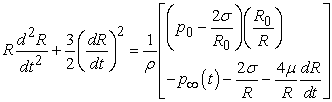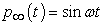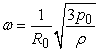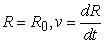﻿ Preliminary Calculation of Bubble Dynamics in Cracking Sound Generation

Preliminary Calculation of Bubble Dynamics in Cracking Sound Generation

Tetsuya Kanagawa, Hisao Taira

Preliminary Calculation of Bubble Dynamics in Cracking Sound Generation

Tetsuya Kanagawa1,, Hisao Taira2

1Department of Engineering Mechanics and Energy, University of Tsukuba, Tsukuba, Japan

2Department of Education, Hokkaido University of Education, Sapporo Campus, Sapporo, Japan

Abstract

Bending a human joint generates a sound. This paper theoretically tackles the cracking sound generated by the manipulation of human joint such as finger. The Rayleigh-Plesset equation in the classical bubble dynamics is applied to determine the nonlinear oscillations of bubble that generates sound. Comparing the numerical solutions of the Rayleigh-Plesset equation for two types of modeling, i.e., forced- and free-oscillator assumptions for inhomogeneous terms, gives clear difference.

• Tetsuya Kanagawa, Hisao Taira. Preliminary Calculation of Bubble Dynamics in Cracking Sound Generation. Biomedical Science and Engineering. Vol. 3, No. 2, 2015, pp 30-34. http://pubs.sciepub.com/bse/3/2/1
• Kanagawa, Tetsuya, and Hisao Taira. "Preliminary Calculation of Bubble Dynamics in Cracking Sound Generation." Biomedical Science and Engineering 3.2 (2015): 30-34.
• Kanagawa, T. , & Taira, H. (2015). Preliminary Calculation of Bubble Dynamics in Cracking Sound Generation. Biomedical Science and Engineering, 3(2), 30-34.
• Kanagawa, Tetsuya, and Hisao Taira. "Preliminary Calculation of Bubble Dynamics in Cracking Sound Generation." Biomedical Science and Engineering 3, no. 2 (2015): 30-34.

 Import into BibTeX Import into EndNote Import into RefMan Import into RefWorks

12
Prev Next

1. Introduction

By bending a joint, we daily recognize the generation of a sound, the so-called cracking sound. The joint such as finger or neck commonly and frequently induces an audible cracking sound. A number of observations [1-7] on cracking sound have intensively been performed and then elucidated the formation of cavitation bubble in the synovial fluid of these joints when a cracking sound is produced. Since the manipulation of joint with cracking sound may damage to the articular capsule or bone [8, 9] we should reveal the origin of the cracking sound because of a medical requirement. The phenomenon is also categorized in the bio-acoustics: The expansion of bubble in the synovial fluid emits a pressure wave, i.e., sound generation. However, the detailed mechanism to explain the cracking sound, especially focusing on a condition necessary to generate an audible sound, has long remained unclear. Hence, many interests have been considerably focused on the audible sound generated by the joint manipulation.

Although many hypotheses based on medical experiments [10, 11, 12] have been proposed, theoretical works from the physical viewpoint have not long been carried out. Minami et al.  clarified that the cracking sound is a complex flow phenomenon accompanied by cavitation  with violent oscillations and destruction of many bubbles. We emphasize that, however, our present target is not to perform a complete numerical simulation considering strongly nonlinear phenomena. In this paper, we theoretically investigate the bubble dynamics with regard to the cracking sound, leading to extract the most important and fundamental physics in the sound. The origin of cracking sound is examined from the viewpoint of fluid mechanics by solving a simple physico-mathematical model, i.e., the Rayleigh-Plesset equation  for the description of bubble oscillations (i.e., contraction and expansion). As a result, the velocity of bubble oscillations readily reaches a sharp peak after the generation of bubble. This tendency represents that the cracking sound is audible at just one time, and coincides with our daily experience regarding joint manipulation.

2. Formulation of the Problem

In the following, we formulate the problem. Let us assume that a single spherical gas bubble is surrounded by an incompressible liquid, and its bubble wall radially oscillates, as sketced in Figure 1. It is well established that the bubble dynamics is described by the following Rayleigh-Plesset equation :(1)

where t is the time, R radius of the bubble, ρ density of the surrounding liquid, p0 hydrostatic pressure, R0 bubble radius in the initial state, γ polytropic index (γ = 1 is used in the following analysis since we consider the isothermal process inside the bubble), μ viscosity of the liquid, σ surface tension coefficient, and p(t) driving pressure. In the right-hand side of Eq. (1), the vapor pressure is dropped. The second term on the left-hand side, (dR/dt)2, represents the second-order nonlinearity. The momentum-conservation equation (or equation of motion) of fluid mechanics  clearly results in the nonlinearity in Eq. (1), i.e., the nonlinear oscillations. Equation (1) describes a forced oscillator (i.e., simple oscillations of the spring-mass system), and its left- and right-hand sides express the inertia and driving forces, respectively.Download asVeiw figureFigures index
Figure 1. Spherically symmetric oscillations of the bubble: R(t) is the bubble radius,  density, p(t) driving pressure, and t time

The driving force acting on the bubble is given by the sinusoidal form:(2)

where ω is the frequency of the driving force. In this paper, we assume that ω equals the eigenfrequency of spherically symmetric linear oscillations of the single bubble, i.e.,(3)

We emphasize that the assumption of Eq. (3) is only for simplicity. Precisely speaking, we should use an eigenfrequency of a coupled-oscillator system composed of the bone, synovial fluid, and bubble, and we substitute it into Eq. (2) instead of Eq. (3). However, this concern contains high difficulty because the dynamical properties of bone and synovial fluid have not fully examined. Nevertheless, as shown in the following result, the set of Eqs. (1)-(3) can predict an essence of the phenomenon because the use of more rigorous formulation of Eq. (3) would not essentially affect our procedure. In the forthcoming report, we will take into account the effect of the coupled oscillation.

Let us solve numerically Eq. (1). The Runge-Kutta scheme with fourth-order central differencing in space has been used, in which the initial conditions are given by(4)

The initial radius R0 was set to 1 μm since R0 should be smaller than the size of the synovial space occupied by the synovial fluid. The initial velocity v was set 0 m/s for simplicity. The values of physical quantities appearing in Eq. (1), i.e., ρ, p0, μ, and σ, are summarized in Table 1. Furthermore, we determined these values by referring to not synovial fluid but water with the normal body temperature of a human. This assumption is intended to easily perform future experiments in order to verify our theoretical prediction.

Table 1. Physical quantities used in the computationDownload asVeiw tableTables index

3. Results and Discussions

Let us discuss the numerical results by considering four cases:

i) free oscillator without external force p(t) as well as surface tension σ,

ii) forced oscillator with external force p(t) and without surface tension σ,

iii) free oscillator without external force p(t) and with surface tension σ,

iv) forced oscillator both with external force p(t) and surface tension σ.

Figure 2(a) and (b) show bubble-wall velocity and radius as a function of time t, respectively in the case of (i).Download asVeiw figureFigures index
Figure 2. Free oscillation without surface tension case (i.e., p(t) = 0, σ = 0): Temporal evolution for (a) bubble wall velocity and (b) bubble radiusDownload asVeiw figureFigures index
Figure 3. Forced oscillation without surface tension case (p(t) is given by Eq. (2), σ = 0): Temporal evolution for (a) bubble wall velocity and (b) bubble radius

The most important observation is that one large and sharp peak appeared around t = 0 for velocity [Figure 2(a)]. This indicates that we recognize the cracking sound only one time and agree with our empirical fact which we generate only one cracking sound by bending our joint. Bubble-wall radius plotted in Figure 2(b) monotonically increases with convex upward. This reveals that the bubble collides with boundary of the synovial space, resulting in the destruction and extinction of the bubble. It would be noted that this extinction theoretically explains the previous experimental result  that bubbles created in the synovial fluid became extinct with time.

In the case of (ii), we take into account the external force p(t), i.e., forced oscillator case but without any surface tension. This is because the external force appearing in Eq. (2) can drives a bubble periodically. We examine the difference between free and forced oscillators. Figure 3(a) and (b) illustrate the bubble-wall velocity and bubble-wall radius versus time t, respectively, on external force. Compare the difference between Figure 2(a) with Figure 3(a). It is clearly seen that amplitude of the bubble-wall velocity reaches a maximum value around t = 0 and subsequently decreasing with time in both figures. The apparent difference is that the functional form of the forced oscillator oscillates but that of free oscillator does not oscillate. This oscillation is originated from the sinusoidal driving force given in Eq. (2). The appearance of multiple peaks in this oscillation implies multiple occurrences of the cracking sound. However, it may hear the sound about two or three times since the amplitude of this oscillation is small except for the region less than t = 0.001 μm. This result indicates that the variation in bubble-wall velocity due to external forces raises the number of the cracking sound. We empirically experience that the cracking sound can be also audible two or three times, thus, in this case, the surface tension is important for the multiply audible sound. The time dependence of the bubble-wall radius [Figure 3(b)] is qualitatively similar to that in bubble-wall velocity [Figure 3(a)]. Because the bubble radii in both Figure 2(b) and Figure 3(b) are roughly the same, for example around t = 0.01 ms, the driving force affects only the small variation in bubble-wall radius. We interpret this property that the time-averaged value of the sinusoidal driving force given in Eq. (2) equals zero.Download asVeiw figureFigures index
Figure 4. Free oscillation with surface tension case (i.e., p(t) = 0, σ = 0.0728 N/m): Temporal evolution for (a) bubble wall velocity and (b) bubble radius

From now on, we discuss the bubble dynamics in cases of (iii) and (iv), i.e., cases of taking into account the surface tension. Figure 4(a) and (b) are counterparts of Figure 2(a) and (b), respectively, but the surface tension is given by σ = 0.0728 N/m, which is the case (iii). The most intriguing characteristics is that both Figure 4(a) and (b) show damped oscillations. We can understand these behaviors by considering the term 2σ/R given in Eq. (1). This term may affect as the damped term. We found that the bubble-wall velocity is large around t = 0. However, the cracking sound may not be audible since the bubble-wall radius is constant value with time, resulting in the bubble does not reaches the boundary of the synovial space and not extinct. This is inconsistent with the previous work . In short, the case (iii) may not be the real situation.Download asVeiw figureFigures index
Figure 5. Forced oscillation with surface tension case (p(t) is given by Eq. (2), σ = 0.0728 N/m): Temporal evolution for (a) bubble wall velocity and (b) bubble radius

Figure 5(a) and (b) are counterparts of Figure 3(a) and (b), respectively, but with the surface tension, which is the case (iv). It is obvious that the cracking sound occurs multiply and this is not consistent with our experience. Thus, the case (iv) as well as the case (iii) may not be the real situation.

4. Conclusions

In this paper, based on the bubble dynamics, the velocity and radius of a single spherical bubble in water have been numerically calculated by using the classical Rayleigh-Plesset equation. We summarize the main results as follows:

Case (i) [p(t) = 0, σ = 0]: A sharp peak in the bubble-wall velocity is appeared. It can be understood that this theoretical prediction validates the empirical observation that the cracking of a joint generates audible sound at only one time.

Case (ii) [p(t) 0, σ = 0]: The cracking sound occurs two or three times. This is also the theoretical validation of audible sounds.

Case (iii) [p(t) = 0, σ = 0.0728 N/m]: The cracking sound may not be audible since the bubble does not extinct with time.

Case (iv) [p(t) 0, σ = 0.0728 N/m]: Multiple occurrences of audible sound are appeared, but this is no realistic. Thus, we can conclude that the cracking sound may be audible when the surface tension is negligibly small in our model.

It is noteworthy that a study based on the physico-mathematical model is absence except for the present study. We expect that the present study will shed light on the qualitative and quantitative understanding of the properties of the cracking sound and will spark the investigation of the condition generating the cracking sound. However, this paper addresses the simplest situation. Further studies are required for aforementioned understanding of the cracking sound, i.e., performing the computation using physical quantities of synovial fluid instead of water, using the eigenfrequency of the coupled-oscillator system composed of the bone, synovial fluid, and bubble, instead of Eq. (3), considering the multiple bubbles and interaction among these bubbles, and incorporating the generation and destruction of bubbles.

References

  J. W. Reggars and M. Chiro, “Multiple channel recording of the articular crack associated with manipulation of the metacarpophalangeal joint. an observational study,” Australas. Chiropr. Osteopat. 8. 16-20. 1999.In article PubMed  M. G. Protapapas and T. C. Cymet, “Joint cracking and popping: Understanding noises that accompany articular release,” J. Am. Osteopath. Assoc. 102. 283-287. 2002.In article PubMed  R. Beffa and R. Mathews, “Does the adjustment cavitate the targeted joint? an investigation into the location of cavitation sounds,” J. Manipulative and Physiol. Ther. 27. 118-122. 2004.In article View Article  PubMed  A. Bolton, R. W. Moran, and C. Standen, “An investigation into the side of joint cavitation associated with cervical spine manipulation,” Int. J. Osteopath. Med. 10. 88-96. 2007.In article View Article  J. Gaetano, “Cracking and cracked knuckle: A medical student’s take,” J. Rheumatology. 36. 2624. 2009.In article View Article  PubMed  K. Weber, M. Olszewski, and R. Ortolano, “Knuckle cracking and hand osteoarthritis,” J. Am. Board Fam. Med. 24. 169-174. 2011.In article View Article  PubMed  J. Dunning, F. Mourad, M. Barbero, D. Leoni, C. Cescon, and R. Butts, “Bilateral and multiple cavitation sounds during upper cervical thrust manipulation,” Musculoskeletal Dis. 14. 16-20. 2013.In article View Article  J. K. Kellgren, J. S. Larence, and F. S. Bier, “Genetic factors in generalized osteoarthrosis,” Ann. Rheum. Dis. 22. 1963.In article  J. Castellanos and D. Axelrod, “Effect of habitual knuckle cracking on hand function,” Ann. Rheum. Dis. 49. 308-309. 1990.In article View Article  PubMed  D. L. Miller, “A review of the ultrasonic bioeffects of microsonation, gas body activation, and related cavitation-like phenomena,” Ultrasound Med. Biol. 13. 443-470. 1987.In article View Article  S. B. Barnett, G. R. ter Haar, M. C. Ziskin, W. L. Nyborg, K. Maeda, and J. Bang, “Current status of research on biophysical effects of ultrasound,” Ultrasound Med. Biol. 20. 205-218. 1994.In article View Article  M. W. Miller, D. L. Miller, and A. A. Brayman, “A review of in vitro bioeffects of inertial ultrasonic cavitation from a mechanistic perspective,” Ultrasound Med. Biol. 22. 1131-1154. 1996.In article View Article  K. Minami, Y. Fukai, T. Kobayashi, Y. Egawa, S. Okada, and H. Ito, Higashi Nihon Seikei Gakkaishi (in Japanese). 16. 333. 2004.In article  M. S. Plesset and A. Prosperetti, “Bubble dynamics and cavitation,” Annu. Rev. Fluid Mech. 9. 145-185. 1977.In article View Article  L. Rayleigh, “On the pressure developed in a liquid during the collapse of a spherical cavity,” Phil. Mag. 34. 94-98. 1919.In article View Article  A. Unsworth, D. Dowson, and V. Wright, “Cracking joints; abioengineering study of cavitation in the metacarpophalangeal joint,” Ann. Rheum. Dis. 30. 345-348. 1971.In article View Article